# Math Tricks v2.6 (Ad-Free) Apk

This program is designed for those who want to learn interesting mathematical tricks to speed up the calculating. These tricks will help ...

This program is designed for those who want to learn interesting mathematical tricks to speed up the calculating. These tricks will help solve part of the mathematical problems and tasks much faster than classical. Will also be helpful to those who want to hone basics such as the multiplication table.
When you learn these mathematical tricks, you'll be able to show off your skills to friends and prove to them that you have a talent for mathematics. New skills you can use in the store, at school, at college, at work - wherever thanks to quick calculation skills can save a lot of precious time.
Fun Math Tricks:
2. Subtraction
3. Multiplication table
4. Division
5. Multiply two digit number by 11
6. Square numbers ending in 5
7. Multiply by 5
8. Multiply by 9
9. Multiply by 4
10. Dividing by 5
11. Subtracting from 1000
12. Tough multiplication
13. Power of two
14. Adding numbers close to hundreds
15. Subtracting numbers close to hundreds
16. Multiply numbers between 11 and 19
17. Square numbers between 11 and 99
18. Multiply two digit numbers having same tens digit and ones digits add up to ten
19. Square numbers between 50 and 59
20. Square numbers between 40 and 49
21. Multiply two digit numbers editing in 1
22. Percent
23. Square numbers between 100 and 109
...
84. n^9
Training mode:
- 15 levels for every trick
- timer 15 seconds
Rating stars:
- score > 000 - ★☆☆
- score > 350 - ★★☆
- score > 650 - ★★★
The app uses the following APIs and libraries:
* MPAndroidChart - created by Philipp Jahoda
* ColorPIcker - created by Piotr Adamus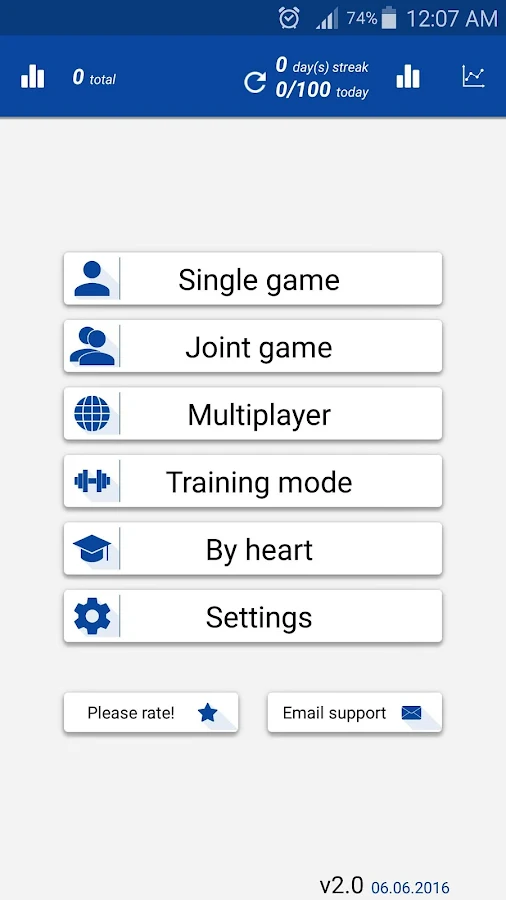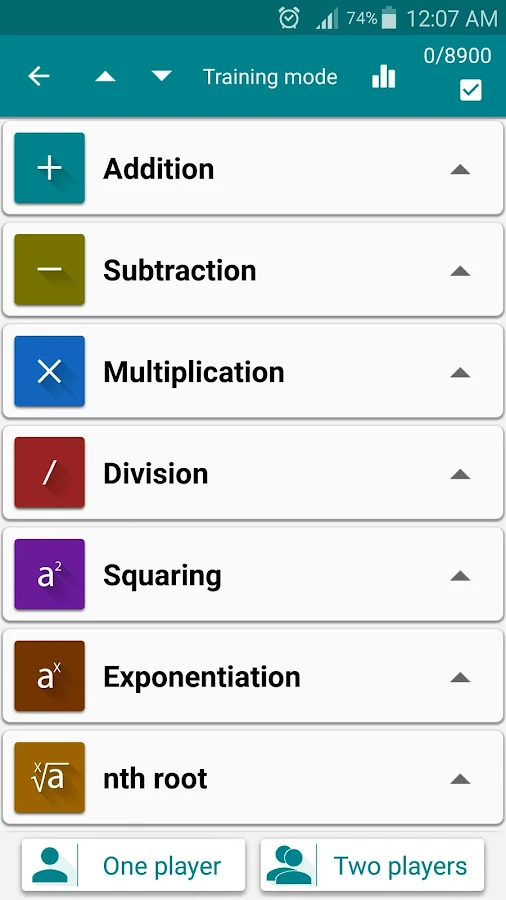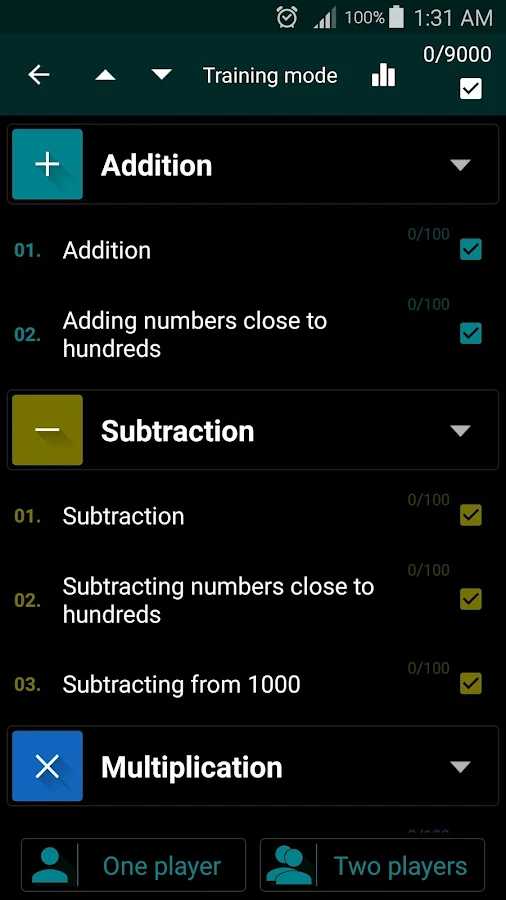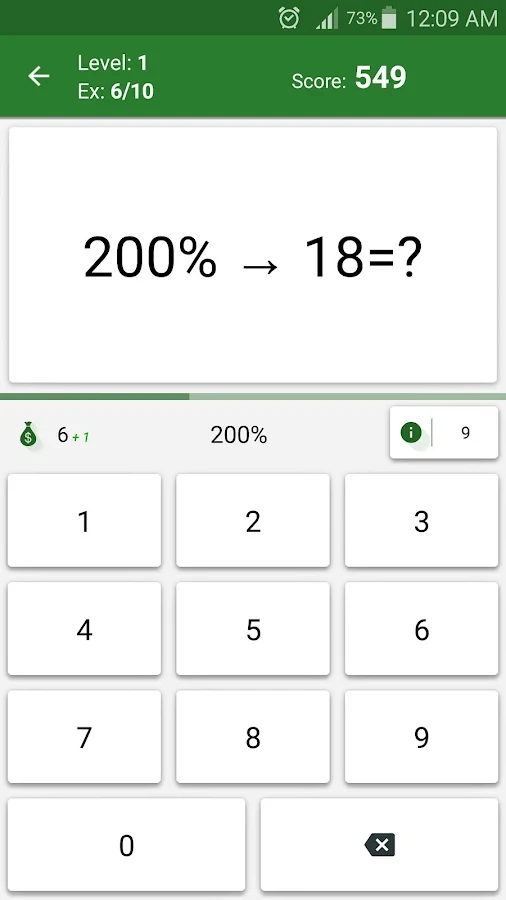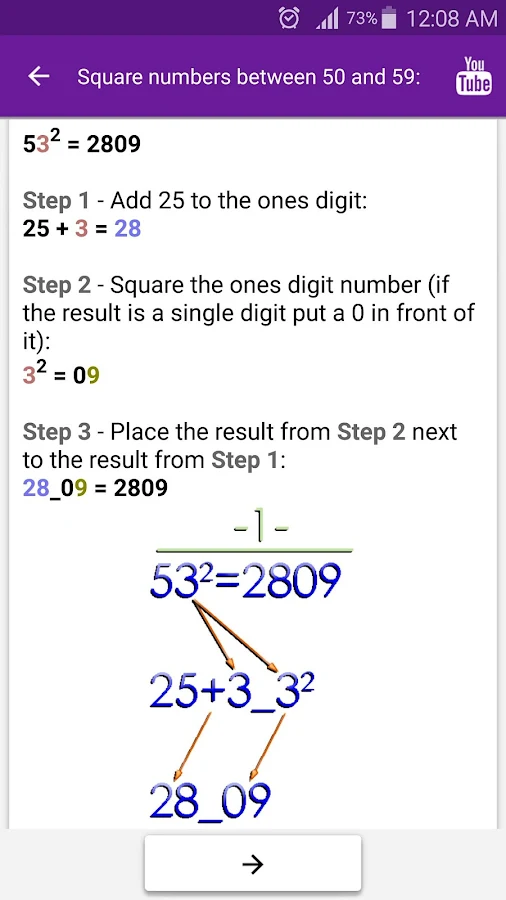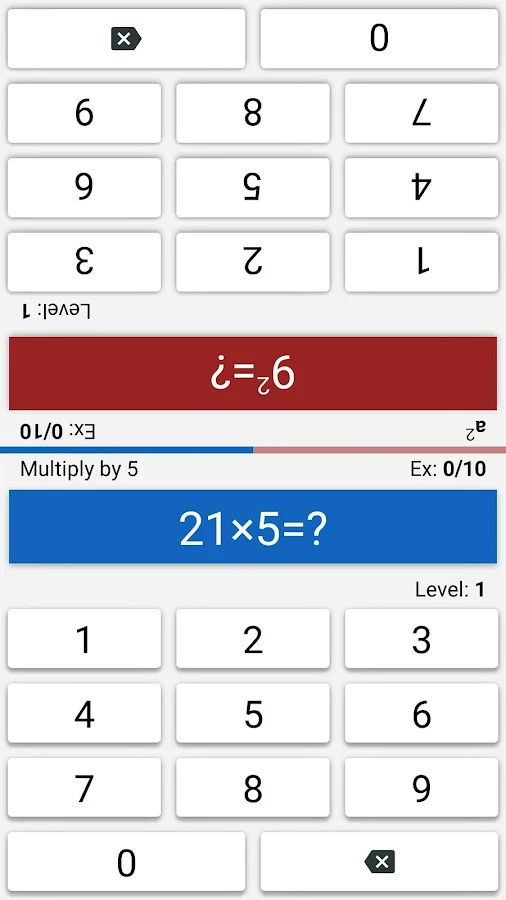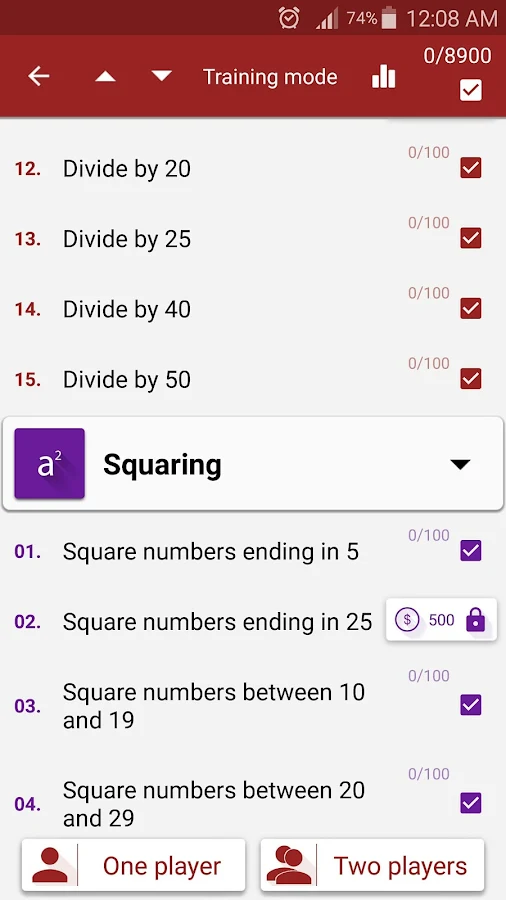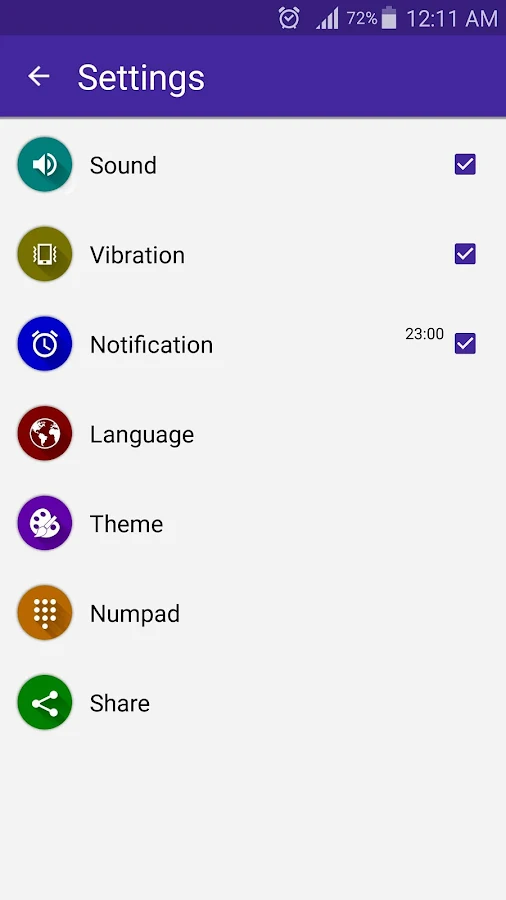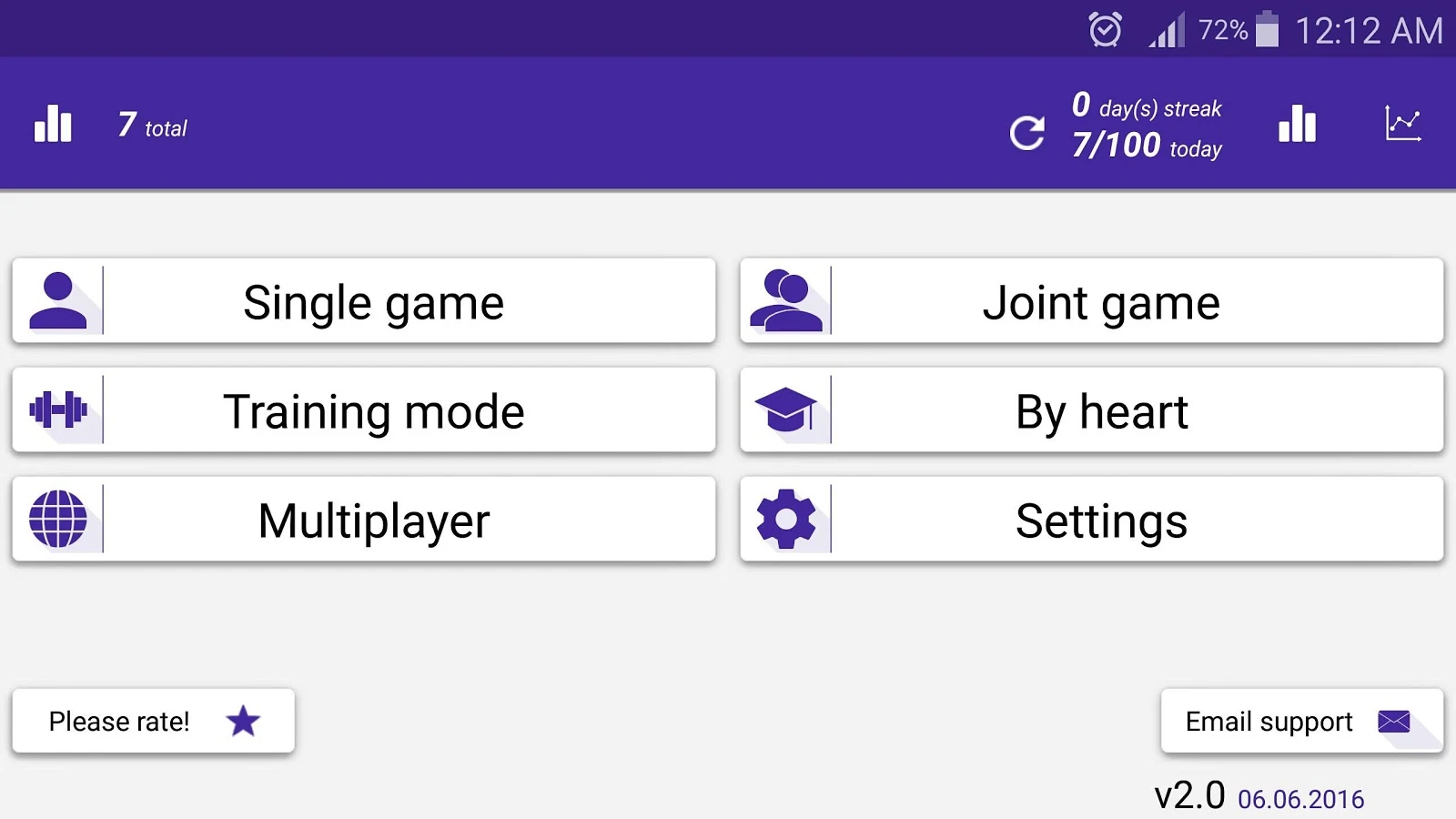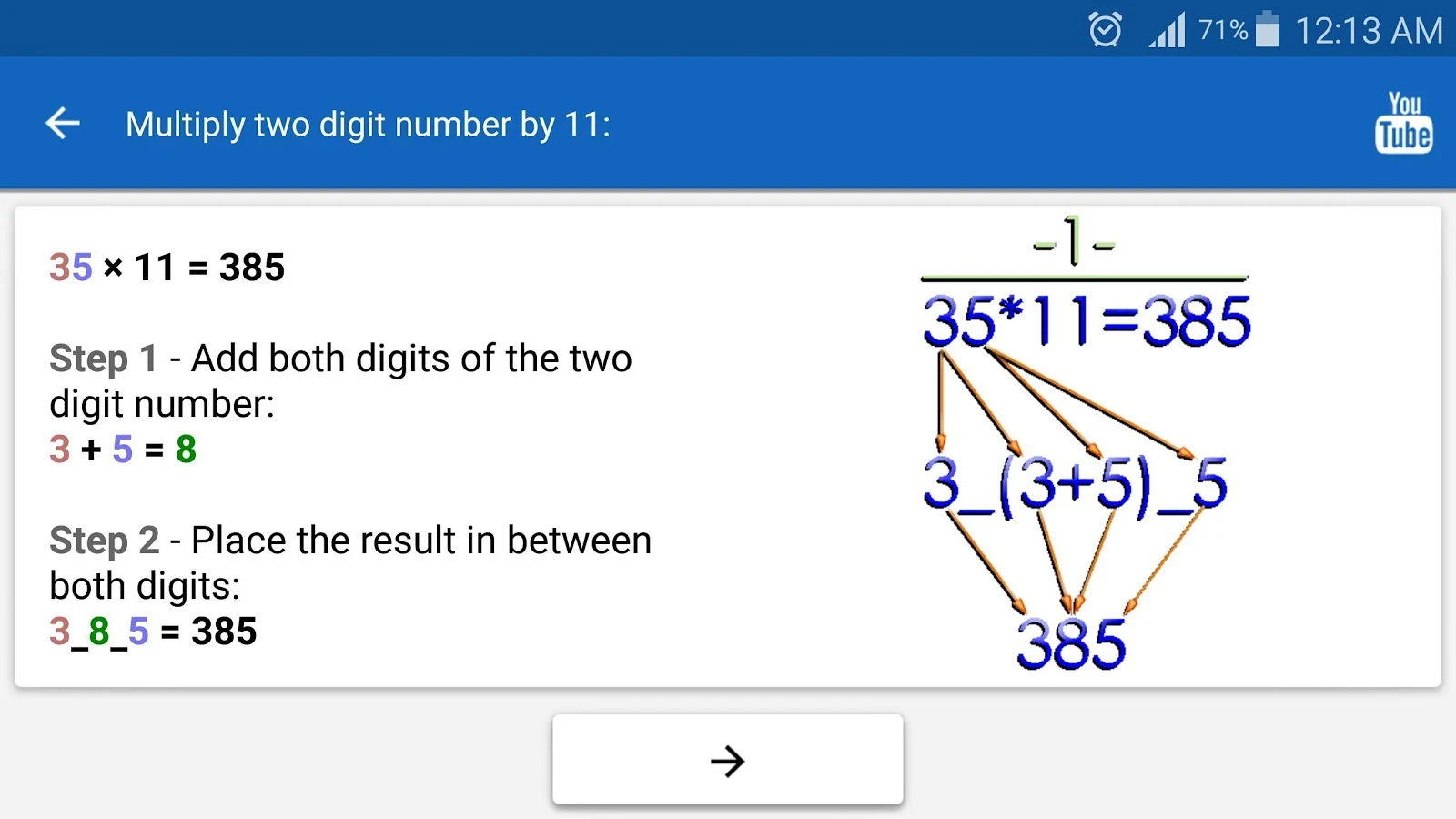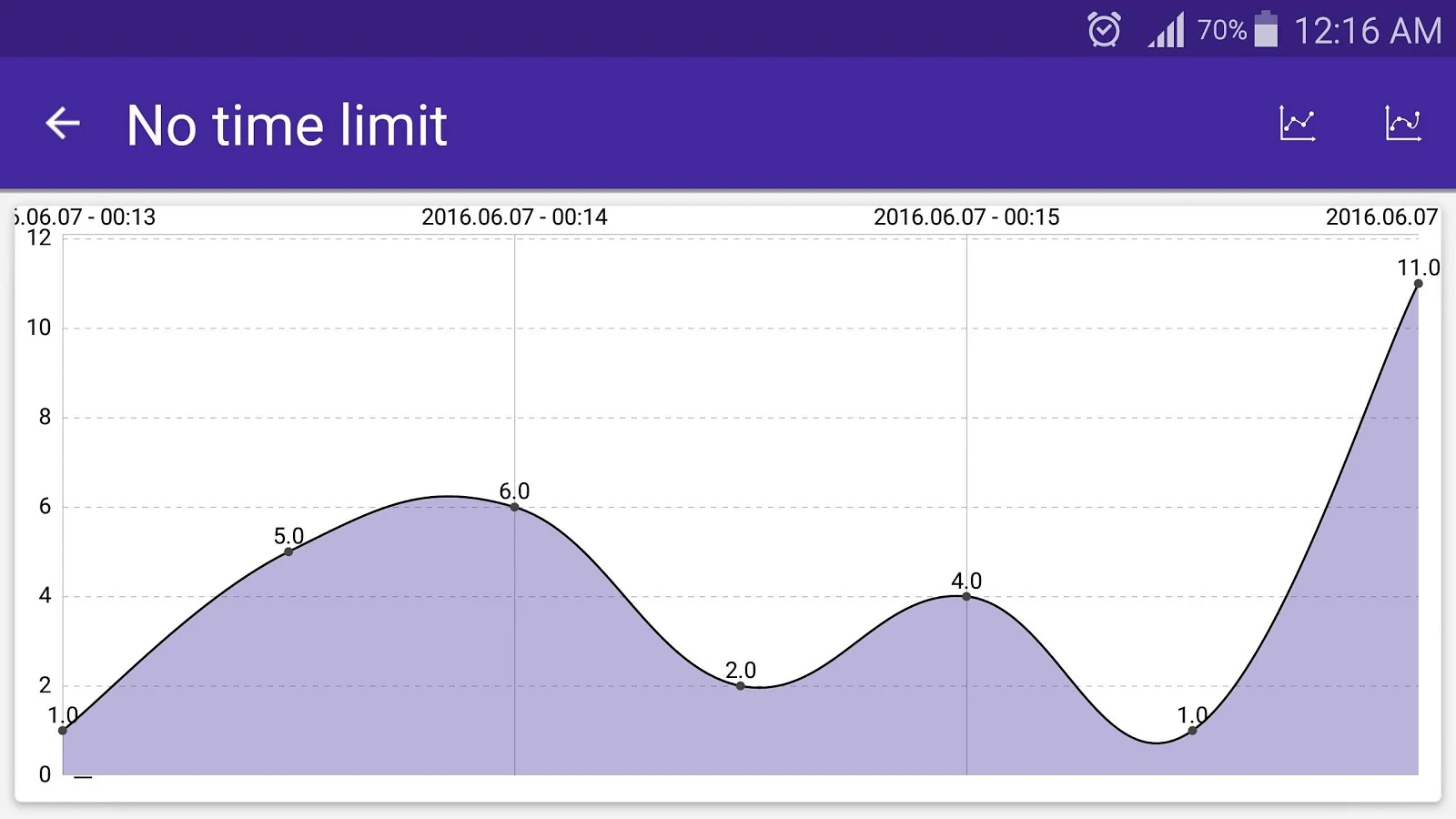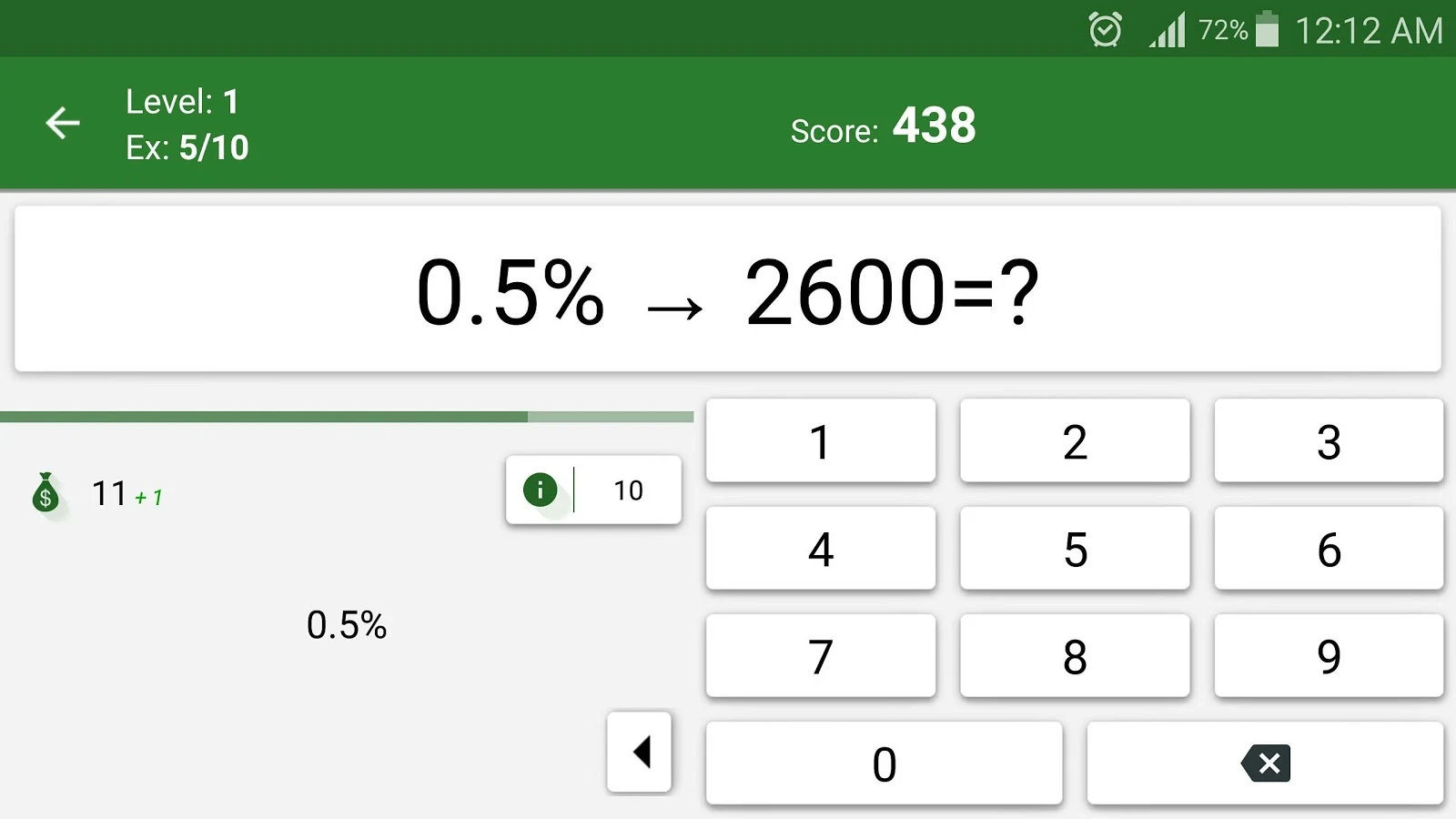# WHAT'S NEW

(As always, keep the feedback coming!) v2.5:

Requires Android2.3 and up